Science

# Kepler’s Laws: All Three

Johannes Kepler, working with data painstakingly collected by Tycho Brahe without the aid of a telescope, developed three laws which described the motion of the planets across the sky.

### 1. Kepler’s Law 1: The Law of Orbits:

All planets move in elliptical orbits, with the sun at one focus.This is the equation for an ellipse:### 2. Kepler’s Law 2: The Law of Areas:

A line that connects a planet to the sun sweeps out equal areas in equal times.### 3. Kepler’s Law 3: The Law of Periods:

The square of the period of any planet is proportional to the cube of the semimajor axis of its orbit.

Ta2 / Tb2 = Ra3 / Rb3

• Square of any planet’s orbital period (sidereal) is proportional to cube of its mean distance (semi-major axis) from Sun
• Mathematical statement: T = kR3/2 , where T = sideral period, and R = semi-major axis
• Example – If a is measured in astronomical units (AU = semi-major axis of Earth’s orbit) and sidereal period in years (Earth’s sidereal period), then the constant k in mathematical expression for Kepler’s third law is equal to 1, and the mathematical relation becomes T2 = R3

### Learn Kepler’s Laws : Live Classroom Video

Kepler’s laws were derived for orbits around the sun, but they apply to satellite orbits as well.

# The Law of Orbits

## All planets move in elliptical orbits, with the sun at one focus.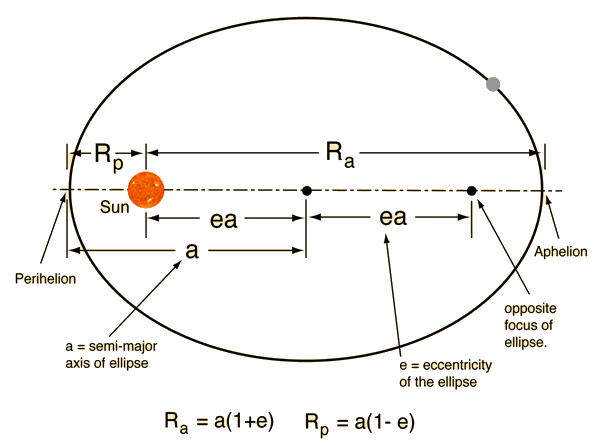This is one of Kepler’s laws. The elliptical shape of the orbit is a result of the inverse square force of gravity. The eccentricity of the ellipse is greatly exaggerated here.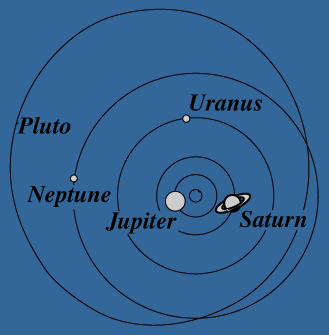# Orbit Eccentricity

The eccentricity of an ellipse can be defined as the ratio of the distancebetween the foci to the major axis of the ellipse. The eccentricity is zero for a circle. Of the planetary orbits, only Pluto has a large eccentricity.

# Examples of Ellipse Eccentricity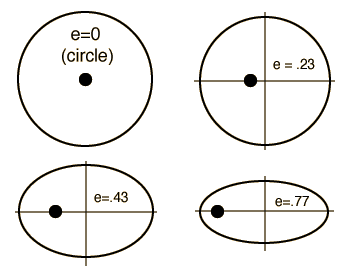Planetary orbit eccentricities

 Mercury 0.206 Venus 0.0068 Earth 0.0167 Mars 0.0934 Jupiter 0.0485 Saturn 0.0556 Uranus 0.0472 Neptune 0.0086 Pluto 0.25

# The Law of Areas

## A line that connects a planet to the sun sweeps out equal areas in equal times.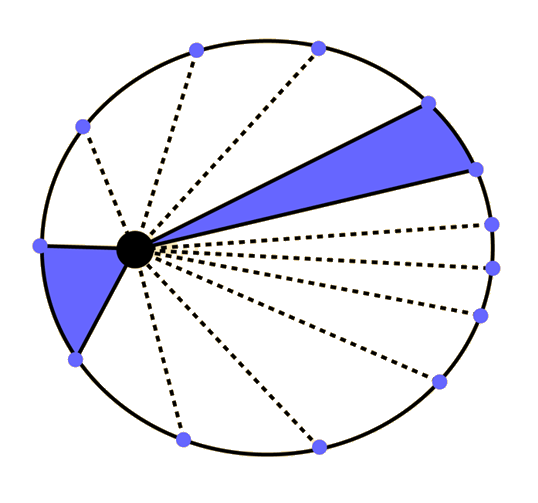This is one of Kepler’s laws.This empirical law discovered by Kepler arises from conservation of angular momentum. When the planet is closer to the sun, it moves faster, sweeping through a longer path in a given time.

# The Law of Periods

## The square of the period of any planet is proportional to the cube of the semimajor axis of its orbit.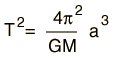This is one of Kepler’s laws.This law arises from the law of gravitation. Newton first formulated the law of gravitation from Kepler’s 3rd law.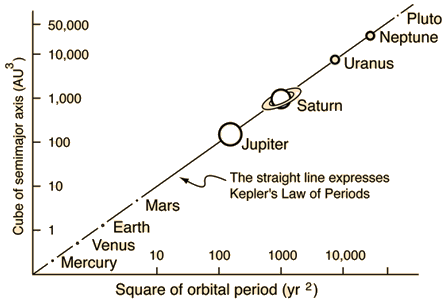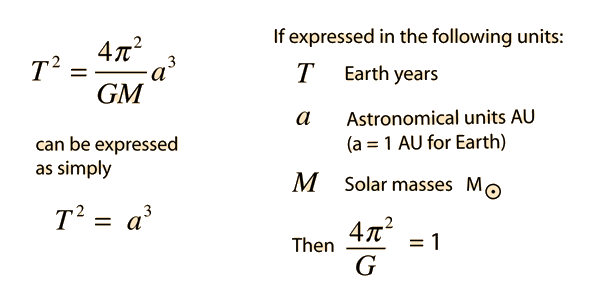Kepler s Law of Periods in the above form is an approximation that serves well for the orbits of the planets because the Sun’s mass is so dominant. But more precisely the law should be written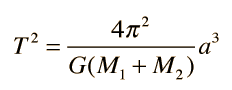In this more rigorous form it is useful for calculation of the orbital period of moons or other binary orbits like those of binary stars.

# Data: Law of Periods

Data confirming Kepler’s Law of Periods comes from measurements of the motion of the planets.

 Planet Semimajor axis (1010m) Period T (y) T2/a3 (10-34y2/m3) Mercury 5.79 0.241 2.99 Venus 10.8 0.615 3.00 Earth 15.0 1 2.96 Mars 22.8 1.88 2.98 Jupiter 77.8 11.9 3.01 Saturn 143 29.5 2.98 Uranus 287 84 2.98 Neptune 450 165 2.99 Pluto 590 248 2.99

Data from Halliday, Resnick, Walker, Fundamentals of Physics 4th Ed Extended.